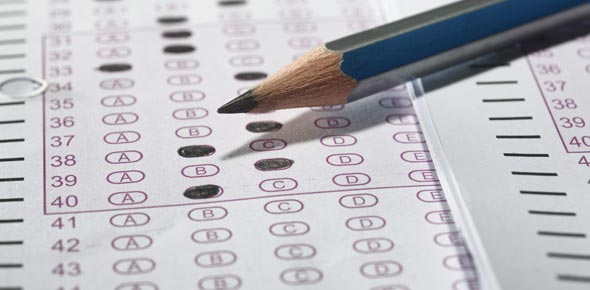# MCQ Set 1 - C

15 Questions | Total Attempts: 141Settings• 1.
What is the output of the following 'C' program? main ( ) { extern int i; i = 20; print ( "% d'', sizeof(i)); }
• A.

2

• B.

4

• C.

Would vary from compiler to compiler

• D.

Error, i undefined

• 2.
Consider the following program: main ( ) { int x = 2, y = 5; if (x < y) return (x = x + y); else print f("zl"); print f("z2"); } Then
• A.

Output is z2

• B.

Output is z1z2

• C.

This will result in compilation error

• D.

None of these

• 3.
What is the output of the following 'C' program? main ( ) { int a  = {2, 3}; print f (''\n % d % d % d'', a , a , a ); }
• A.

Garbage values

• B.

2 3 3

• C.

3 2 2

• D.

0 0 0

• 4.
What is the output of the following 'C' program? main ( ) { int i = 2; print f (''\n % d % d'', + + i, + + i); }
• A.

3 4

• B.

4 3

• C.

4 4

• D.

Output may vary from compiler to compiler

• 5.
What is the output of the following 'C' program? main ( ) { int x = 10, y = 10, z = 5, i; i = x <y <z; printf ("\n % d'', i); }
• A.

1

• B.

0

• C.

Error

• D.

5

• 6.
In the following 'C' code in which order the functions would be called? a = (f 1 (23, 14) * f 2 (12 / 4) + f 3 ( ));
• A.

F1, f2, f3

• B.

F3, f2, f1

• C.

The order may vary from compiler to compiler

• D.

None of these

• 7.
What is the output of the following 'C' program? main { Float a = 0. 7; if (a < 0.7) print f (''C''); else print f ('' C + + ''); }
• A.

C

• B.

C++

• C.

Error

• D.

None of the above

• 8.
What is the output of the following 'C' program? main ( ) { printf ('' % f '', sqrt (36.0)); }
• A.

6.0

• B.

6.000000

• C.

6

• D.

Some absurd result

• 9.
We want to round off x, a float, to an int value. What is the correct way to do so?
• A.

Y = (int) (x + 0.5);

• B.

Y = int (x +0.5);

• C.

Y = (int) x + 0.5;

• D.

Y = (int (int) x + 0.5;

• 10.
By default any real number in 'C' is treated as
• A.

A float

• B.

A double

• C.

A long double

• D.

Depends upon the memory model that you are using

• 11.
What is the output of the following 'C' program? main ( ) { printf (''% d % d % d", sizeof(3.14 f), sizeof (3.14), sizeof (3.14 l)); }
• A.

4 4 4

• B.

4 Garbage value

• C.

4 8 10

• D.

Error

• 12.
The following program main ( ) { static int a { } = {7, 8, 9}; print f("%d", a {2} = 2 [a] + A ; }
• A.

Results in bus error

• B.

Results in segmentation violation error

• C.

Will not compile successfully

• D.

None of these

• 13.
What is the output of the following 'C' program? main { printf (''%c'', ''abcdefgh'' );}
• A.

Error

• B.

D

• C.

E

• D.

Abcdefgh

• 14.
What is the output of the following 'C' program? main ( ) { char str  = ''strings''; print ('' % c ''; str); }
• A.

Error

• B.

Strings

• C.

Cannot predict

• D.

None of these

• 15.
Which header file should you include if you are to develop a function that can accept variable number of arguments?
• A.

Vararg. h

• B.Back to top